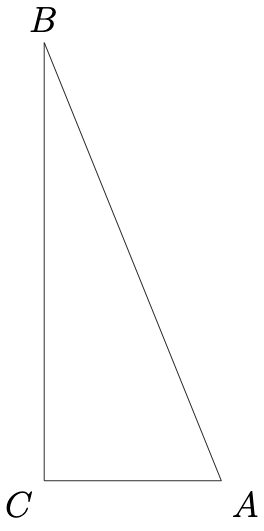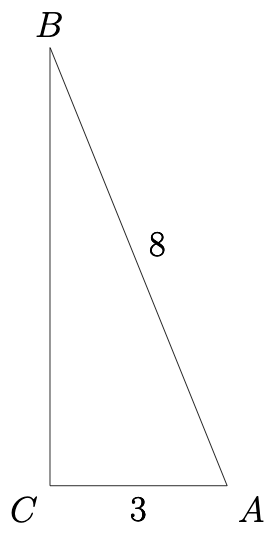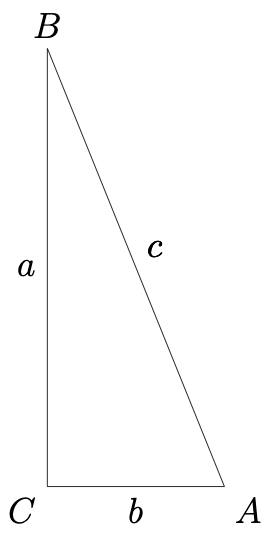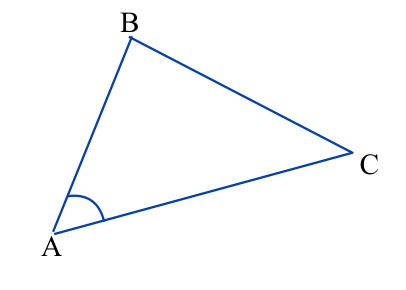## Finding an Angle’s Sine Given the Sine of Its Complement

This post about finding an angle’s sine given the sine of its complement is part of a series of posts to help you prepare for the Advanced Algebra and Functions part of the Accuplacer test.

## Question

Triangle ABC is a right triangle. Sin B = 3/8. What is the value of sin A?

## Solution

“Sin” is an abbreviation for sine. The sine of angle A in a right triangle means the ratio of the length of the side opposite angle A to the length of the hypotenuse (longest side).

You are asked to find an angle’s sine given the sine of its complement. A diagram should help. We can make some guesses about how triangle ABC looks. It’s conventional to name the right angle of a right triangle C, so right triangle ABC may look like this:Right triangle ABCRight triangle ABC.

That the sine of angle B is 3/8 means the length of the side opposite angle divided by the length of the hypotenuse is 3/8.

Those lengths may be 3 and 8 (or 30 and 80 or 0.3 and 0.8 or any combination in the proportion of 3/8).

The sine of angle is equal to the length of the side opposite angle A divided by the length of the hypotenuse. What is the length of the side opposite angle A?

### Sidebar: Pythagorean Theorem.

The Pythagorean Theorem states that the square of the length of the hypotenuse of a right triangle equals the sum of the squares of the lengths of the legs.Right triangle ABC

In other words, using the labels in the triangle at left, where each side is named for the angle opposite it, .

### Back to the Question

We need to know a. Solve the Pythagorean equation above for a:

And we know that and . Then

Now the triangle looks like this:Right triangle ABC.

The sine of A is the length of side opposite A divided by the length of the hypotenuse:

This question is similar to question number 20 in the sample questions for the Accuplacer Advanced Algebra and Functions test.

## Congruent Triangles

This post about congruent triangles is part of a series of posts to help you prepare for the Advanced Algebra and Functions part of the Accuplacer test.

## Question

Angle A in triangle ABC is congruent to angle D in triangle DEF. Which of the statements below, if it’s true, proves that triangles ABC and DEF are congruent?

A.  and

B.   and

C.   and

D.   andTriangle DEF.Triangle ABC.

## Solution

Two triangles are congruent if one of these things is true:

• every side of one is congruent to a side of the other (SSS);
• two sides and the angle between them in one are congruent to two sides and the angle between them in the other (SAS); or
• two angles and any side in one are congruent to two angles and any side of the other (ASA or AAS).

Let’s go through the answer choices to see if one of them meets one of those criteria.Triangle DEF

A.  and .Triangle ABC

That’s  two  angles and the side between them in one triangle being congruent to the corresponding parts in the other triangle. That’s enough to meet the first criterion above, so it looks like that’s the answer. But let’s check the others.

B.   andTriangle ABC.

That’s two sides and an angle, but not the angle between them, so it does not do the job.Triangle DEF

C.   and .  Same problem as answer choice B: Two sides and one angle of one are congruent to two sides and one angle of the other, but the angle is not the one between the two sides, so that information is not enough for a proof.Triangle DEFTriangle ABC

D.  and . This gives you only congruent angles. Every proof of congruent triangles requires at least one side.Triangle ABC